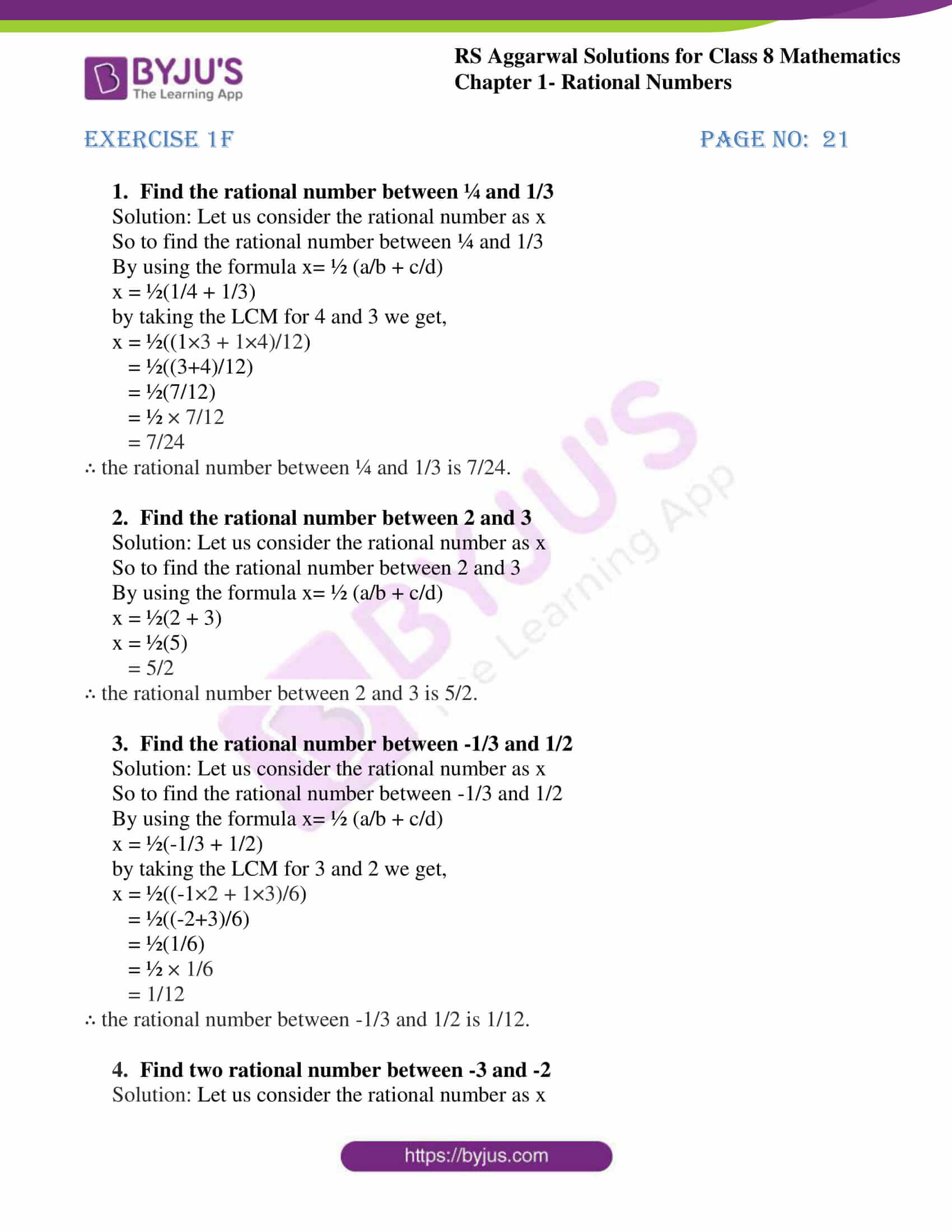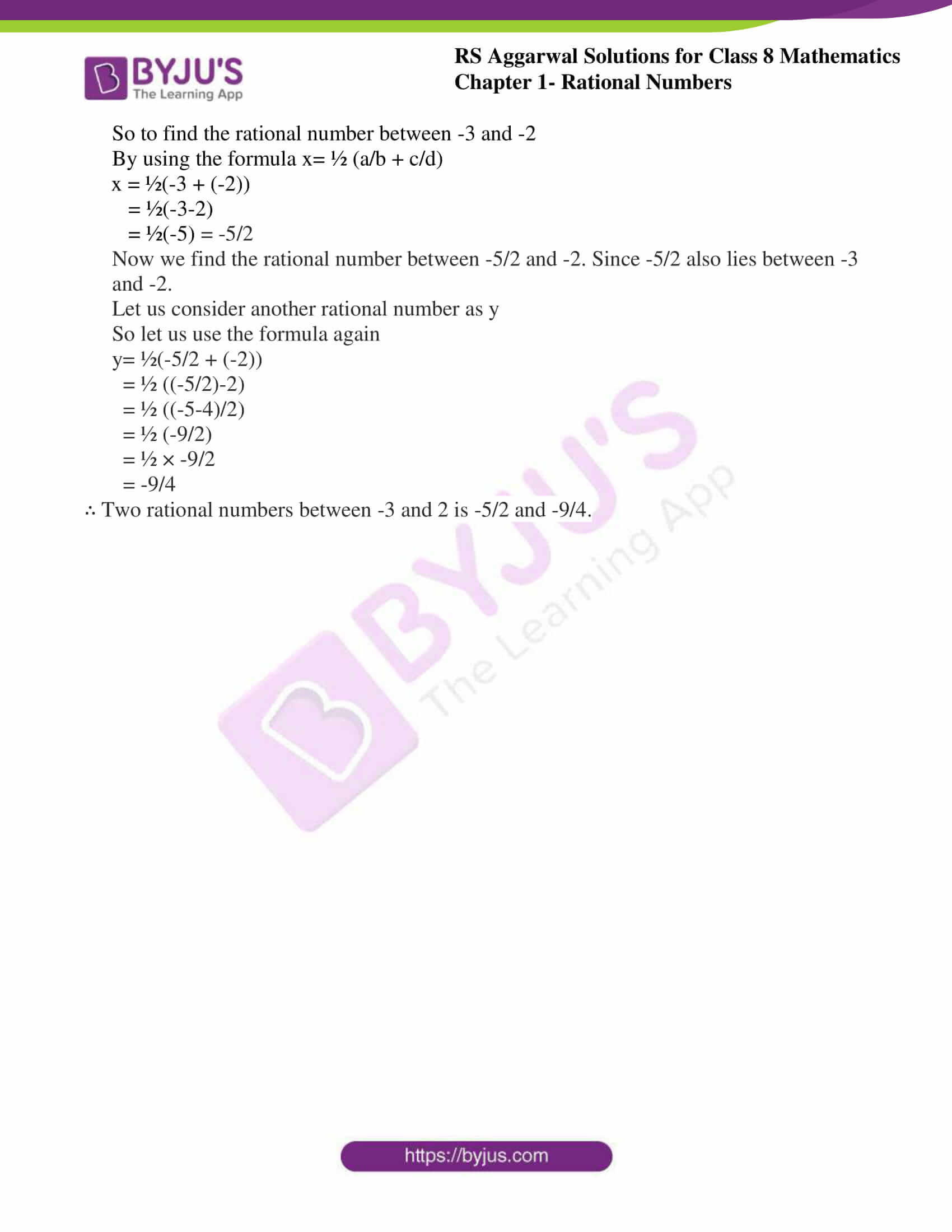# RS Aggarwal Solutions for Class 8 Maths Chapter 1 - Rational Numbers Exercise 1F

BYJU’S expert team has provided RS Aggarwal Solutions for Class 8 Maths Chapter 1- Exercise 1F, Rational Numbers, downloaded from the links provided below. Students are suggested to practice the RS Aggarwal Solutions problems in order to be thorough with their concepts.

In Exercise 1F of RS Aggarwal Class 8 Maths, we shall study the alternative method of finding a large number of Rational Numbers between two given Rational Numbers.

## Download PDF of RS Aggarwal Solutions for Class 8 Maths Chapter 1- Rational Numbers Exercise 1F### Access Answers to RS Aggarwal Solutions for Class 8 Maths Chapter 1- Rational Numbers Exercise 1F

1. Find the rational number between ¼ and 1/3

Solution: Let us consider the rational number as x

So to find the rational number between ¼ and 1/3

By using the formula x= ½ (a/b + c/d)

x = ½(1/4 + 1/3)

by taking the LCM for 4 and 3 we get,

x = ½((1×3 + 1×4)/12)

= ½((3+4)/12)

= ½(7/12)

= ½ × 7/12

= 7/24

∴ the rational number between ¼ and 1/3 is 7/24.

2. Find the rational number between 2 and 3

Solution: Let us consider the rational number as x

So to find the rational number between 2 and 3

By using the formula x= ½ (a/b + c/d)

x = ½(2 + 3)

x = ½(5)

= 5/2

∴ the rational number between 2 and 3 is 5/2.

3. Find the rational number between -1/3 and 1/2

Solution: Let us consider the rational number as x

So to find the rational number between -1/3 and 1/2

By using the formula x= ½ (a/b + c/d)

x = ½(-1/3 + 1/2)

by taking the LCM for 3 and 2 we get,

x = ½((-1×2 + 1×3)/6)

= ½((-2+3)/6)

= ½(1/6)

= ½ × 1/6

= 1/12

∴ the rational number between -1/3 and 1/2 is 1/12.

4. Find two rational number between -3 and -2

Solution: Let us consider the rational number as x

So to find the rational number between -3 and -2

By using the formula x= ½ (a/b + c/d)

x = ½(-3 + (-2))

= ½(-3-2)

= ½(-5) = -5/2

Now we find the rational number between -5/2 and -2. Since -5/2 also lies between -3 and -2.

Let us consider another rational number as y

So let us use the formula again

y= ½(-5/2 + (-2))

= ½ ((-5/2)-2)

= ½ ((-5-4)/2)

= ½ (-9/2)

= ½ × -9/2

= -9/4

∴ Two rational numbers between -3 and 2 is -5/2 and -9/4.

### Access other Exercises of RS Aggarwal Solutions for Class 8 Maths Chapter 1- Rational Numbers

Exercise 1A Solutions 10 Questions

Exercise 1B Solutions 3 Questions

Exercise 1C Solutions 14 Questions

Exercise 1D Solutions 9 Questions

Exercise 1E Solutions 12 Questions

Exercise 1G Solutions 20 Questions

Exercise 1H Solutions 23 Questions

## RS Aggarwal Solutions for Class 8 Maths Chapter 1- Rational Numbers Exercise 1F

Exercise 1F of RS Aggarwal Class 8 Chapter 1, Rational Numbers contains the advance concepts related to Rational Numbers. This exercise mainly deals with finding the larger number between any two given Rational Numbers.

The RS Aggarwal textbook provides solutions that are very helpful for the students to practice which yields good marks. Practicing as many times as possible helps in time management skills and also boosts the confidence level to achieve your goals.